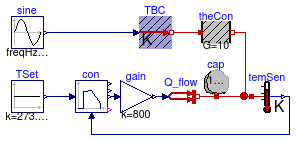## Buildings.Controls.Continuous.Examples

Collection of models that illustrate model use and test models

### Information

This package contains examples for the use of models that can be found in Buildings.Controls.Continuous.

Extends from Modelica.Icons.ExamplesPackage (Icon for packages containing runnable examples).

### Package Content

NameDescriptionLimPID Test model for PID controller with optional reverse actionPIDHysteresis Example model for PID controller with hysteresisPIDHysteresisTimer Example model for PID controller with hysteresis and timerSignalRanker Example model for signal rankerNumberOfRequests Example model for block that outputs the number of requestsOffTimer Example model for off timer

##Buildings.Controls.Continuous.Examples.LimPID

Test model for PID controller with optional reverse action### Information

This model tests the implementation of the PID controller with optional reverse action. The model `limPIDOri` is the original implementation of the controller from the Modelica Standard Library. The models `limPID` and `limPIDRev` are the implementations from the Buildings library. The model `limPIDRev` is parameterized to have reverse action. The assertion blocks test whether the results of all three controllers are identical.

Extends from Modelica.Icons.Example (Icon for runnable examples).

### Modelica definition

```model LimPID
"Test model for PID controller with optional reverse action"
extends Modelica.Icons.Example;

Modelica.Blocks.Sources.Pulse pulse(period=0.25);
Buildings.Controls.Continuous.LimPID limPID(
controllerType=Modelica.Blocks.Types.SimpleController.PID,
Ti=1,
Td=1,
yMax=1,
yMin=-1,
initType=Modelica.Blocks.Types.InitPID.InitialState);
Buildings.Controls.Continuous.LimPID limPIDRev(
controllerType=Modelica.Blocks.Types.SimpleController.PID,
reverseAction=true,
Ti=1,
Td=1,
yMax=1,
yMin=-1,
initType=Modelica.Blocks.Types.InitPID.InitialState)
"Controller with reverse action";
Modelica.Blocks.Sources.Constant const(k=0.5);
Modelica.Blocks.Math.Gain gain(k=-1);
Buildings.Utilities.Diagnostics.AssertEquality assertEquality(threShold=1e-10);
Modelica.Blocks.Continuous.LimPID limPIDOri(
controllerType=Modelica.Blocks.Types.SimpleController.PID,
Ti=1,
Td=1,
yMax=1,
yMin=-1,
initType=Modelica.Blocks.Types.InitPID.InitialState);
Buildings.Utilities.Diagnostics.AssertEquality assertEquality1(
threShold=1e-10);
equation
connect(pulse.y, limPID.u_s);
connect(pulse.y, limPIDRev.u_s);
connect(const.y, limPID.u_m);
connect(const.y, limPIDRev.u_m);
connect(limPIDRev.y, gain.u);
connect(gain.y, assertEquality.u2);
connect(limPID.y, assertEquality.u1);
connect(pulse.y, limPIDOri.u_s);
connect(const.y, limPIDOri.u_m);
connect(assertEquality1.u1, limPIDOri.y);
connect(assertEquality1.u2, limPID.y);
end LimPID;
```

##Buildings.Controls.Continuous.Examples.PIDHysteresis

Example model for PID controller with hysteresis### Information

Example that demonstrates the use of the PID controller with hysteresis. The control objective is to keep the temperature of the energy storage `cap` at 40°C. The controller `con` is parameterized to switch on if the control error is bigger than eon=1. The output of the controller remains above ymin=0.3 until the control error is smaller than eoff=-1, at which time the controller outputs y=0 until the control error is again bigger than 1. The figure below shows the control error `con.feeBac.y` and the control signal `con.y`.Extends from Modelica.Icons.Example (Icon for runnable examples).

### Modelica definition

```model PIDHysteresis
"Example model for PID controller with hysteresis"
extends Modelica.Icons.Example;

Buildings.Controls.Continuous.PIDHysteresis con(
pre_y_start=false,
controllerType=Modelica.Blocks.Types.SimpleController.PI,
yMin=0.3,
Ti=600,
Td=60);
Modelica.Blocks.Sources.Constant TSet(k=273.15 + 40, y(unit="K")) "Set point";
Modelica.Thermal.HeatTransfer.Components.HeatCapacitor cap(C=1000000, T(start=
313.15, fixed=true));
Buildings.HeatTransfer.Sources.PrescribedTemperature TBC;
Modelica.Thermal.HeatTransfer.Components.ThermalConductor theCon(G=20);
Modelica.Blocks.Math.Gain gain(k=2000);
Modelica.Thermal.HeatTransfer.Sensors.TemperatureSensor temSen;
Buildings.HeatTransfer.Sources.PrescribedHeatFlow Q_flow;
Modelica.Blocks.Sources.Sine sine(
freqHz=1/86400,
offset=273.15,
amplitude=20,
phase=-1.5707963267949,
y(unit="K"));
equation

connect(TSet.y, con.u_s);
connect(TBC.port, theCon.port_a);
connect(theCon.port_b, cap.port);
connect(con.y, gain.u);
connect(cap.port, temSen.port);
connect(temSen.T, con.u_m);
connect(gain.y, Q_flow.Q_flow);
connect(Q_flow.port, cap.port);
connect(sine.y, TBC.T);
end PIDHysteresis;
```

##Buildings.Controls.Continuous.Examples.PIDHysteresisTimer

Example model for PID controller with hysteresis and timer### Information

Example that demonstrates the use of the PID controller with hysteresis and off timer. The example is identical to Buildings.Controls.Continuous.Examples.PIDHysteresis, except that the controller also has an off timer. This timer keeps the control signal at y=0 for a period of `minOffTime=1000` seconds. This may be used to avoid short-cycling if the load is small and the system has little heat capacity.

The figure below shows the control error `con.feeBac.y` and the control signal `con.y`.Extends from Modelica.Icons.Example (Icon for runnable examples).

### Modelica definition

```model PIDHysteresisTimer
"Example model for PID controller with hysteresis and timer"
extends Modelica.Icons.Example;

Buildings.Controls.Continuous.PIDHysteresisTimer con(
yMin=0.3,
minOffTime=10000,
controllerType=Modelica.Blocks.Types.SimpleController.PI,
Ti=60,
Td=10);
Modelica.Blocks.Sources.Constant TSet(k=273.15 + 40, y(unit="K")) "Set point";
Modelica.Thermal.HeatTransfer.Components.HeatCapacitor cap(C=100000, T(start=
293.15, fixed=true));
Buildings.HeatTransfer.Sources.PrescribedTemperature TBC;
Modelica.Thermal.HeatTransfer.Components.ThermalConductor theCon(G=10);
Modelica.Blocks.Math.Gain gain(k=800);
Modelica.Thermal.HeatTransfer.Sensors.TemperatureSensor temSen;
Buildings.HeatTransfer.Sources.PrescribedHeatFlow Q_flow;
Modelica.Blocks.Sources.Sine sine(
freqHz=1/86400,
offset=273.15,
amplitude=20,
phase=-1.5707963267949);
equation

connect(TSet.y, con.u_s);
connect(TBC.port, theCon.port_a);
connect(theCon.port_b, cap.port);
connect(con.y, gain.u);
connect(cap.port, temSen.port);
connect(temSen.T, con.u_m);
connect(gain.y, Q_flow.Q_flow);
connect(Q_flow.port, cap.port);
connect(sine.y, TBC.T);
end PIDHysteresisTimer;
```

##Buildings.Controls.Continuous.Examples.SignalRanker

Example model for signal ranker### Information

Example that demonstrates the use of the signal ranker model. The figure below shows the input and output signals of the block. Note that `sigRan.y ≥ sigRan.y ≥ sigRan.y`.Extends from Modelica.Icons.Example (Icon for runnable examples).

### Modelica definition

```model SignalRanker "Example model for signal ranker"
extends Modelica.Icons.Example;
Modelica.Blocks.Sources.Sine sine(freqHz=2);
Modelica.Blocks.Sources.Pulse pulse(period=0.25);
Buildings.Controls.Continuous.SignalRanker sigRan(
nin=3);
Modelica.Blocks.Sources.ExpSine expSine(freqHz=10, damping=1);
equation
connect(sine.y, sigRan.u);
connect(pulse.y, sigRan.u);
connect(expSine.y, sigRan.u);
end SignalRanker;
```

##Buildings.Controls.Continuous.Examples.NumberOfRequests

Example model for block that outputs the number of requests### Information

Example that demonstrates the use of the block Buildings.Controls.Continuous.NumberOfRequests. The parameters of the block are such that the output is incremented for each input signal that is strictly larger than 0. The figure below shows the inputs and the output of the block.Extends from Modelica.Icons.Example (Icon for runnable examples).

### Modelica definition

```model NumberOfRequests
"Example model for block that outputs the number of requests"
extends Modelica.Icons.Example;
Buildings.Controls.Continuous.NumberOfRequests numReq(
nin=2,
threShold=0,
kind=0);
Modelica.Blocks.Sources.Sine sine(freqHz=2);
Modelica.Blocks.Sources.Pulse pulse(period=0.25);
equation
connect(sine.y, numReq.u);
connect(pulse.y, numReq.u);
end NumberOfRequests;
```

##Buildings.Controls.Continuous.Examples.OffTimer

Example model for off timer### Information

Example that demonstrates the use of the model Buildings.Controls.Continuous.OffTimer. The input to the two timers are alternating boolean values. Whenever the input becomes `false(=0)`, the timer is reset. The figures below show the input and output of the blocks.Extends from Modelica.Icons.Example (Icon for runnable examples).

### Modelica definition

```model OffTimer "Example model for off timer"
extends Modelica.Icons.Example;

Modelica.Blocks.Sources.BooleanPulse booleanPulse(period=0.2);
Buildings.Controls.Continuous.OffTimer offTim1;
Buildings.Controls.Continuous.OffTimer offTim2;
Modelica.Blocks.Logical.Not not1;
equation
connect(booleanPulse.y, offTim1.u);
connect(booleanPulse.y, not1.u);
connect(offTim2.u, not1.y);
end OffTimer;
```

Automatically generated Thu Oct 24 15:08:05 2013.